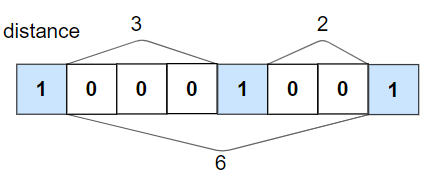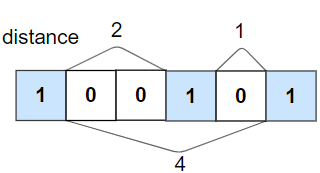@TOC

# # 题目描述``````输入：nums = [1,0,0,0,1,0,0,1], k = 2

````````````输入：nums = [1,0,0,1,0,1], k = 2

``````

``````输入：nums = [1,1,1,1,1], k = 0

``````

``````输入：nums = [0,1,0,1], k = 1

``````

1. `1 <= nums.length <= 10^5`
2. `0 <= k <= nums.length`
3. `nums[i]` 的值为 `0``1`

# # 解题方法

## # 指针

Python 代码如下：

``````class Solution(object):
def kLengthApart(self, nums, k):
"""
:type nums: List[int]
:type k: int
:rtype: bool
"""
left_1 = float("-inf")
for i, num in enumerate(nums):
if num == 1:
if i - left_1 < k + 1:
return False
left_1 = i
return True
``````

# # 日期

2020 年 5 月 3 日 —— 天气好热，瞬间入夏